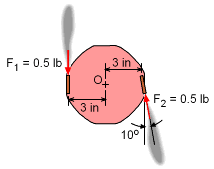Ch 4. Moments/Equivalent Systems Multimedia Engineering Statics Moment2-D Scalar Moment3-D Scalar Moment3-D Vector Couples and Equiv. System Distributed Loads, Intro
 Chapter 1. Basics 2. Vectors 3. Forces 4. Moments 5. Rigid Bodies 6. Structures 7. Centroids/Inertia 8. Internal Loads 9. Friction 10. Work & Energy Appendix Basic Math Units Sections Search eBooks Dynamics Fluids Math Mechanics Statics Thermodynamics Author(s): Kurt Gramoll ©Kurt GramollSTATICS - CASE STUDY IntroductionForce directions acting on the misaligned firework Several types of fireworks use two rockets to cause the fireworks to spin rapidly and rise up in the air. If the thrust from the two rockets is not aligned properly, the fireworks will move in a certain direction as well as spin. This direction can be determined from an equivalent force-couple system or force resultant. What is known: Each rocket has a thrust of T = 0.5 lb. One of the engines is misaligned by α = 10°. The necessary dimensions are shown. Questions What is the equivalent force-couple system at point O? If one is possible, what is the force resultant? Approach Use a vector approach to determine the equivalent force and moment at point O. Solve for the position of the force resultant, if one is possible.

Practice Homework and Test problems now available in the 'Eng Statics' mobile app
Includes over 500 problems with complete detailed solutions.
Available now at the Google Play Store and Apple App Store.